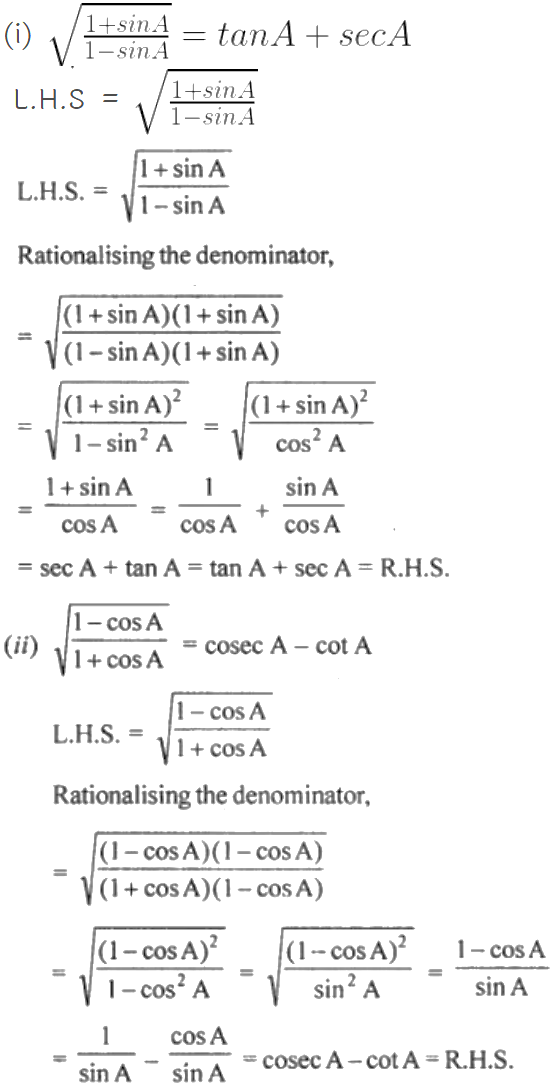Guru

# (i) Root{(1+sinA)/(1-sinA)}= tanA+secA (ii) root{(1-cosA)/(1+cosA)}= cosecA-cotA

• 0

This is an important question from the
Book- ML Aggarwal
Board- ICSE
Publication- Avichal
Chapter- Trigonometric Identities
Chapter number-18

We have to prove the given equality

(i) Root{(1+sinA)/(1-sinA)}= tanA+secA (ii) root{(1-cosA)/(1+cosA)}= cosecA-cotA
Trigonometric Identities, class 10th, chapter 18th, question no 23, ICSE board, ML aggarwal..

Share